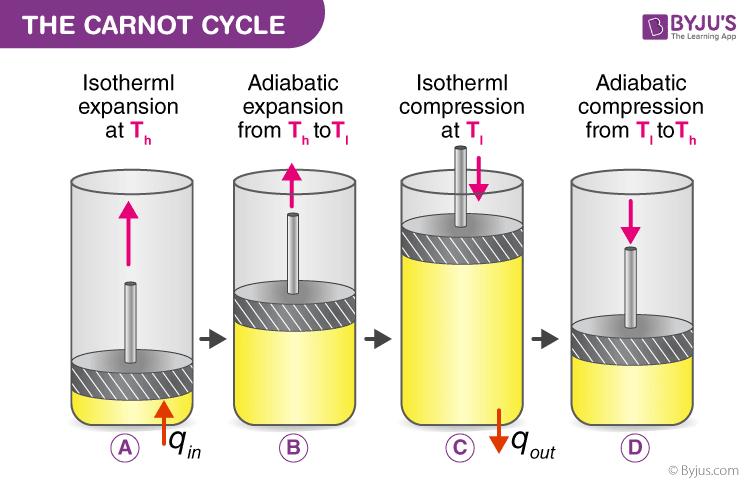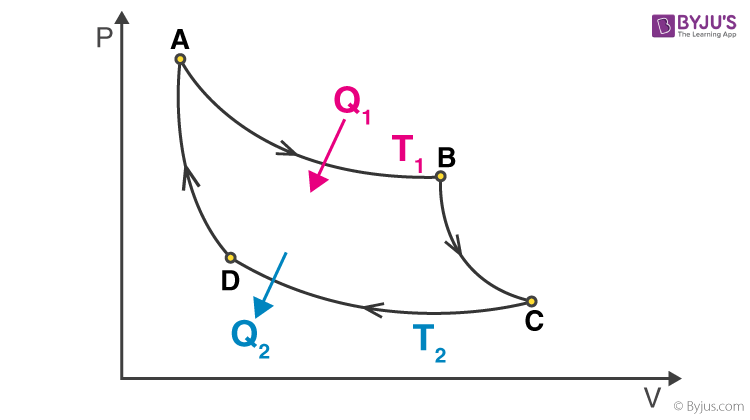# Carnot Engine - Thermodynamic Engine

A Carnot engine is a theoretical engine that operates on the Carnot cycle. Nicolas Leonard Sadi Carnot developed the basic model for this engine in 1824. In this descriptive article, you will learn about the Carnot cycle and Carnot Theorem in detail.

## What is Carnot Engine?

Carnot engine is a theoretical thermodynamic cycle proposed by Leonard Carnot. It estimates the maximum possible efficiency that a heat engine during the conversion process of heat into work and, conversely, working between two reservoirs can possess.

### Carnot Theorem

According to Carnot Theorem:

Any system working between T1 (hot reservoir) and T2 (cold reservoir) can never have more efficiency than the Carnot engine operating between the same reservoirs.

Also, the efficiency of this type of engine is independent of the nature of the working substance and is only dependent on the temperature of the hot and cold reservoirs.

## Carnot Cycle

A Carnot cycle is defined as an ideal reversible closed thermodynamic cycle. Four successive operations are involved: isothermal expansion, adiabatic expansion, isothermal compression, and adiabatic compression. During these operations, the expansion and compression of the substance can be done up to the desired point and back to the initial state.Following are the four processes of the Carnot cycle:

• In (a), the process is reversible isothermal gas expansion. In this process, the amount of heat absorbed by the ideal gas is qin from the heat source at a temperature of Th. The gas expands and does work on the surroundings.
• In (b), the process is reversible adiabatic gas expansion. Here, the system is thermally insulated, and the gas continues to expand and work is done on the surroundings. Now the temperature is lower, Tl.
• In (c), the process is reversible isothermal gas compression process. Here, the heat loss qout occurs when the surroundings do the work at temperature Tl.
• In (d), the process is reversible adiabatic gas compression. Again the system is thermally insulated. The temperature again rises back to Th as the surrounding continue to do their work on the gas.

## Steps involved in a Carnot Cycle

For an ideal gas operating inside a Carnot cycle, the following are the steps involved:

### Step 1:

Isothermal expansion: The gas is taken from P1, V1, T1 to P2, V2, T2. Heat Q1 is absorbed from the reservoir at temperature T1. Since the expansion is isothermal, the total change in internal energy is zero, and the heat absorbed by the gas is equal to the work done by the gas on the environment, which is given as:

$$W_{1\rightarrow 2}=Q1=\mu \times R\times T_{1}\times ln\frac{v_{2}}{v_{1}}$$

### Step 2:

Adiabatic expansion: The gas expands adiabatically from P2, V2, T1 to P3, V3, T2.

Here, work done by the gas is given by:

$$W_{2\rightarrow 3}=\frac{\mu R}{\gamma -1}(T_{1}-T_{2})$$

### Step 3:

Isothermal compression: The gas is compressed isothermally from the state (P3, V3, T2) to (P4, V4, T2).

Here, the work done on the gas by the environment is given by:

$$W_{3\rightarrow 4}=\mu RT_{2}ln\frac{v_{3}}{v_{4}}$$

### Step 4:

Adiabatic compression: The gas is compressed adiabatically from the state (P4, V4, T2) to (P1, V1, T1).

Here, the work done on the gas by the environment is given by:

$$W_{4\rightarrow 1}=\frac{\mu R}{\gamma -1}(T_{1}-T_{2})$$Hence, the total work done by the gas on the environment in one complete cycle is given by:

$$W= W_{1\rightarrow 2}+W_{2\rightarrow 3}+W_{3\rightarrow 4}+W_{4\rightarrow 1}\\ \\ W=\mu \: RT_{1}\: ln\frac{v_{2}}{v_{1}}-\mu \: RT_{2}\: ln\frac{v_{3}}{v_{4}}$$

$$Net\; efficiency =\frac{Net\; workdone\; by\; the\;gas}{Heat\; absorbed\; by\; the\;gas}$$

$$Net\; efficiency =\frac{W}{Q_{1}}=\frac{Q_{1}-Q_{2}}{Q_{1}}=1-\frac{Q_{2}}{Q_{1}}=1-\frac{T_{2}}{T_{1}}\frac{ln\frac{v_{3}}{v_{4}}}{ln\frac{v_{2}}{v_{1}}}$$

Since the step 2–>3 is an adiabatic process, we can write T1V2Ƴ-1 = T2V3Ƴ-1

Or,

$$\frac{v_{2}}{v_{3}}=(\frac{T_{2}}{T_{1}})^{\frac{1}{\gamma -1}}$$

Similarly, for the process 4–>1, we can write

$$\frac{v_{1}}{v_{2}}=(\frac{T_{2}}{T_{1}})^{\frac{1}{\gamma -1}}$$

This implies,

$$\frac{v_{2}}{v_{3}}=\frac{v_{1}}{v_{2}}$$

So, the expression for net efficiency of Carnot engine reduces to:

$$Net\; efficiency=1-\frac{T_{2}}{T_{1}}$$

## Frequently Asked Questions – FAQs

### What is a working fluid in Carnot’s cycle?

The working fluid in a Carnot’s cycle is an ideal gas.

### What is a Carnot heat engine?

Carnot heat engine is a theoretical engine that operates on a reversible carnot cycle. It has maximum efficiency that a heat engine can possess.

### Name the processes involved in Carnot cycle?

It involves four process: isothermal expansion, adiabatic expansion, isothermal compression and adiabatic compression.

### What happens in the isothermal expansion process?

In the isothermal expansion process, gas is taken from P1, V1, T1 to P2, V2, T2. Heat Q1 is absorbed from the reservoir at temperature T1. The total change in internal energy is zero and the heat absorbed by the gas is equal to the work done.

### Is Carnot’s cycle reversible?

The Carnot heat-engine cycle described is a totally reversible cycle.

Watch the video and understand how thermodynamic processes are applied to heat engines.Test your knowledge on Carnot engine

Great

2. Amit Dey

What is the success of the Carnot engine?

1. Carnot Engine is 64% efficient.

1. The efficiency of a Carnot heat engine is given by the Formula: 1 – T2/T1, which has been derived above.
Thus the efficiency will completely depend on the temperatures of the source and the sink and is not a constant, However for a given set of source temperature and sink temperature the Carnot heat engine is the most efficient engine possible.

3. Supreeth s

Nice explanation! Thank you!